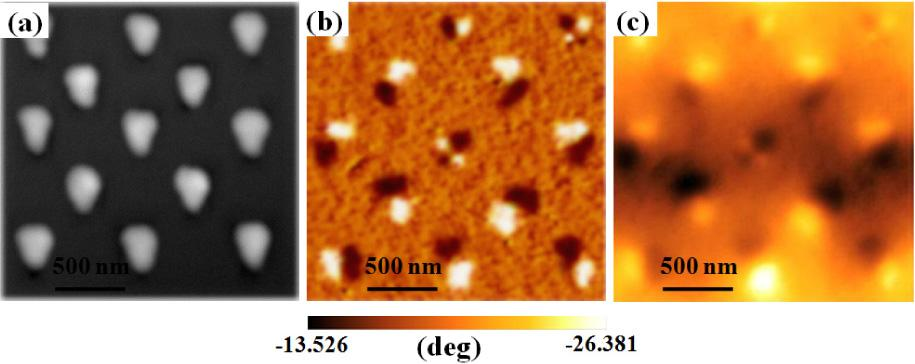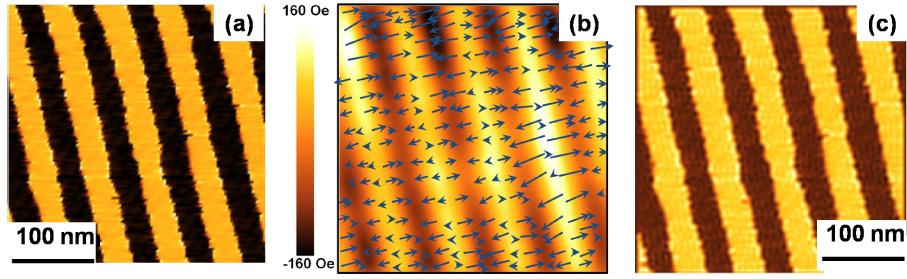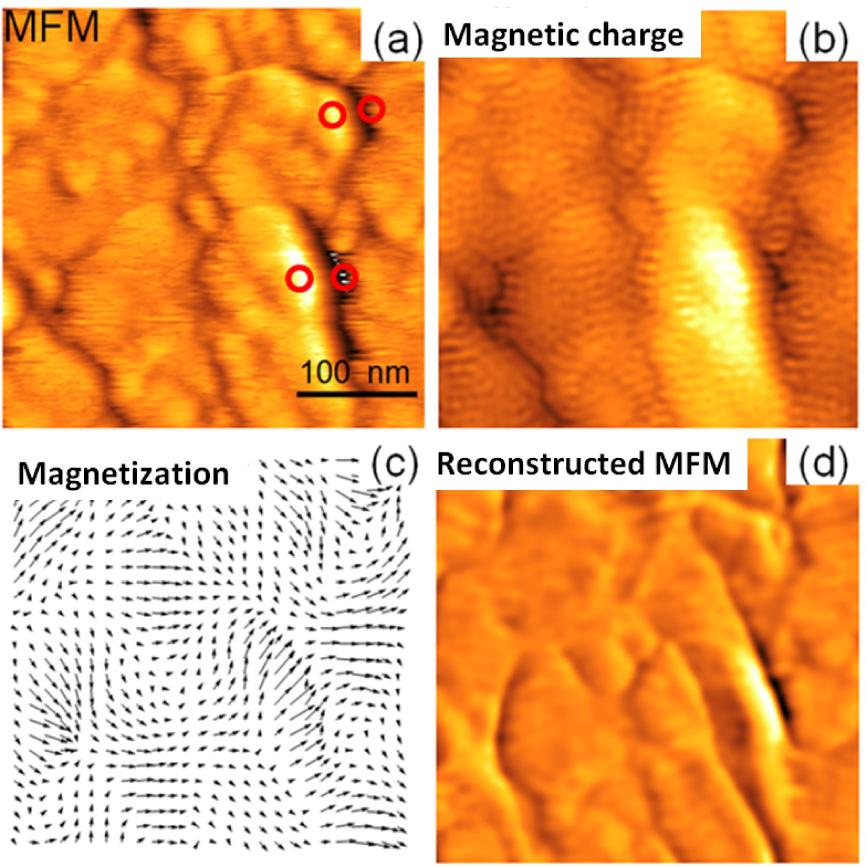Journal DetailsFormat
Journal
eISSN
2083-134X
First Published
16 Apr 2011
Publication timeframe
4 times per year
Languages
English
Open Access

# A new method for quantifying the nanoscale magnetic domains

###### Accepted: 30 Oct 2016
Journal DetailsFormat
Journal
eISSN
2083-134X
First Published
16 Apr 2011
Publication timeframe
4 times per year
Languages
English

In this work, a signal transformation method to quantitatively analyze fine magnetic structures in nanometer length scales measured by magnetic force microscopy has been developed. Nanosized magnetic domains, magnetic charges with reconstructed polarity as well as quantified magnetic field contours of samples (such as ordered FePt dot arrays, hard magnetic thin films and polycrystalline La0.7Sr0.3 MnO3 (LSMO) films ) were investigated based on the basic principles of deconvolution and micromagnetics. The present technique is crucial for the analysis of fine magnetic structures, and is important for the development of next generation magnetic recording industry.

#### Keywords

Introduction

Recently, understanding the nature of nanoscale magnetic domain structures and applying their unique magnetic properties in a wide range of magnetic commercial products, such as hard disk drives (HDD), spin based devices and so on, underpins the development of next generation magnetic storage technologies . As the magnetic recording density further increases (future ultrahigh density target is of 100 TB/in2), the typical bit size will be reduced to several nanometers. Therefore, the understanding and quantifying the fine magnetic structures in nanometer length scales is of great importance for the development of the next generation magnetic commercial products.

Magnetic force microscopy (MFM) is a stateof-the-art tool used to image the nanoscale magnetic domains in a wide range of magnetic nanostructures [5, 6]. For investigating the surface of fine magnetic structures in magnetic nanostructures, the conventional MFM can only qualitatively explain the magnetic stray field originating from the sample surface. How to quantify the MFM images and discover the detailed magnetic moments distributions in samples, has been considered as an urgent problem raised in MFM techniques due to its potential applications in a wide range of areas, such as data storage, biomedicine, and so on.

In this work, a singnal transformation algorithm to quantify magnetic domains in ordered FePt dot arrays, hard magnetic thin films (CoCrPt) and polycrystalline La0.7Sr0.3MnO3 (LSMO) films was developed. The nanosized magnetic domains, the related magnetic charge distribution, as well as the magnetic field contours of the samples have been investigated based on the basic principles of deconvolution and micromagnetics. The good agreement between the simulated and experimental results reveals that the present method is effective for analyzing the microscopic magnetic domain states in a variety of magnetic materials.

Experimental

The microscopic magnetic domain configurations of the samples were imaged by using a conventional MFM system in air atmosphere. The lift height of the MFM tip was varied from 20 nm to 40 nm. The resonant frequency of the tip was approximately 256 kHz. The samples were the ordered FePt dot arrays, hard magnetic thin films (CoCrPt) and polycrystalline Lao0.7Sro0.3MnO3 (LSMO) films. The measurements were implemented using an ultra sharp and high coercivity FePt tip. For the analysis of the fine magnetic structures, the high coercivity FePt tip was optimized and magnetized perpendicular to the sample surface. The MFM tip behavior was assumed as a magnetic monopole.

Theory

The MFM phase signal can be actually considered as a convolution of surface magnetic charge and magnetic field from the MFM probe tip: $Fz′(r)=∫r′σ(r′)Tρ(r−r′)dr′$$$F_z'(r)= \int\limits_{r'} \sigma (r')T_\rho (r-r') dr'$$

where F′z(r) is the magnetic force gradient perpendicular to sample surface,σr′ is the surface magnetic charge distribution, Tρ(r - r′) is the transformation function of the tip corresponding to a point magnetic charge.

Since the MFM tip behavior can be assumed as a magnetic monopole, and assuming that the tip-to-sample distance is constant during the scanning process, the transformation function of the assumed monopole tip can be analytically obtained in the Fourier space : $Tρ(kx,ky,z)=12π∫−∞+∞Tρ(k)eikzzdkz=−πqtμ0kx2+ky2e−kx2+ky2z$$$\begin{array}{l}{T_\rho (k_x, k_y, z)} & = \frac {1}{{2}\pi}\int\limits_{-\infty}^{+\infty}{T_\rho} (k)e^{i{k_z}z}d{k_z}\\ & = - \frac{\pi {q}_{t}}{{{\mu _{{0}}}}} \sqrt {k_x^2 + k_y^2} {e^{-\sqrt {k_x^2{+}k_y^2 z} }}\end{array}$$

where Tρ(kx, ky, z) represents the tip transformation function in the Fourier domain, µ0 is the permeability of vacuum, qt is the magnetic charge of a monopole type tip.

Based on equation 1, the magnetic charge distribution can be obtained based on the principle of de-convolution: $σ(r) = F−1Fz′(kr)Tρ (kx,ky,z) = σmax⋅σi(x,y)$ $$\sigma (r){\text{ = }}{F^{ - 1}}\left\{ {\frac{{F_z' ({k_r})}}{{{T_\rho }{\text{ (}}{k_x},{k_y}, z{\text{)}}}}} \right\}{\text{ = }}{\sigma _{\max }} \cdot {\sigma _i}(x,y)$$

where: F′Z(kr) is the magnetic force gradient in the Fourier space, σmax is the maximum charge density at the sample surface, σi denotes the normalized surface magnetic charge distribution. ρmax can be obtained by using the measured M-H loop of the samples.

In the micromagnetic modeling (the simulation program was developed by our research group using FORTRAN language), the total energy in each grid includes the Zeeman energy, crystalline anisotropy energy, shape anisotropy energy, exchange interaction, and the magnetostatic interaction . In the micromagnetic simulation, the reconstructed surface magnetic charge can be used as the input parameter to drive the magnetic domain reversal. The fine magnetic domain structures and their related magnetization reversal process can be evaluated based on the principles of Landau-Lifshitz-Gilbert (LLG) equations.

Results and discussion

Fig. 1 shows (a) the AFM image, (b) the MFM phase image, and (c) the quantified magnetic field distributions of the triangular shaped FePt pad with in-plane magnetization. The lift height of the tip is 20 nm. Fig. 1a clearly shows the AFM morphology of the individual dots within the array. Fig. 1b shows the MFM phase image for the longitudinally oriented FePt dot arrows. Based on Fig. 1b, it can be observed that the highest intensity is captured at the edge, or near the vertex of the triangular shape. The MFM phase image clearly shows a characteristic pattern with dark-bright dipole MFM contrast, indicating that the dot arrays should be in a single or multiple domain state with magnetization parallel to one edge of the triangular dot. Fig. 1c shows quantified magnetic field contours of the triangular shaped FePt pad obtained by using the developed signal transformation algorithm on the detected MFM signal (Fig. 1b). Based on the results of Fig. 1, we can clearly observe the distribution of magnetic field (Fig. 1c) as well as the magnetic field gradient (Fig. 1b) emanated from the dot arrays, both of which are of great importance for designing the magnetic nanostructures.(a) the AFM image, (b) the MFM phase image, and (c) the quantified magnetic field distribution of the triangular shaped FePt pad with in-plane magnetization.

Fig. 2a shows the MFM phase image for a perpendicular magnetic recording media. The MFM phase image shows characteristic stripes with dark-bright MFM contrast, and the recorded bits can be clearly distinguished by the two-valued contrast. Fig. 2b shows the quantified in-plane and out-ofplane magnetic field distributions near the sample surface. The magnetic field can be obtained from the dependence: $H→(r→)=∑i=1nρmax4πμ0ρi(r′→)(r→−r′→)r→−r′→3$$$\mathop {\overrightarrow H (\overrightarrow r ) = \sum\limits_{i = 1}^n {\frac{{\rho_\max }}{{4\pi {\mu _0}}}} \frac{{{\rho _i}(\overrightarrow {r'}) (\overrightarrow r - \overrightarrow {r'} )}}{{{{\left| {\overrightarrow r - \overrightarrow {r'}} \right|}^3}}}}$$(a) The MFM phase image, (b) the quantified perpendicular and in-plane magnetic field components, (c) the simulated MFM image for the perpendicular magnetic recording media.

The color in Fig. 2b shows the magnitude and direction of the perpendicular component (z component, Hz) of magnetic field, while the vectors represent the direction and value of in-plane components of surface magnetic field (the value varies between 0 to 7162 A/m). From the results it is clearly seen that the features of quantified magnetic field distributions (Fig. 2b) are totally different from those of the measured MFM phase image (Fig. 2a showing sharp edges). Because the MFM phase image is proportional to the field gradients, therefore, the big difference can be well characterized as the z-component magnetic field contour (Hz) with respect to its gradient $∂Hz∂z$ $\frac{{\partial {\text{Hz}}}}{{\partial {\text{z}}}}$ . Fig. 2c shows the simulated MFM image based on the quantified results of Fig. 2b. The reconstructed MFM image can be obtained by calculating the field gradient ($∂Hz∂z$ $\frac{{\partial {\text{Hz}}}}{{\partial {\text{z}}}}$ ) from the film surface by using the micromagnetic method. It is found that the reconstructed result agrees well with the measured MFM image (Fig. 2a).

Fig. 3 shows (a) MFM, (b) magnetic charge distribution, (c) magnetization distribution and (d) reconstructed magnetic field gradient ($∂Hz∂z$ $\frac{{\partial {\text{Hz}}}}{{\partial {\text{z}}}}$ ) of the granular La0.7Sr0.3MnO3 (LSMO) thin film. The sample is in the magnetized state, the magnetization lies in the film plane. The phase detection image (a) can be considered as the z-component magnetic field gradient above the film surface, and the MFM image shows a characteristic pattern with dark-bright features (marked by red circles) near the grain boundaries with nonmagnetic phase. The results reveal that the studied LSMO granular films are magnetized parallel to the applied field direction, causing the surface magnetic charges to concentrate mainly at the nonmagnetic boundaries. Fig. 3b shows the quantified magnetic charge distribution of the LSMO film based on the principles of deconvolution method. The result clearly shows that the reconstructed magnetic charges favor to concentrate at the nonmagnetic boundaries, which is consistent with the experimental analysis. Fig. 3c shows the reconstructed magnetization distribution of the studied samples magnetized in the film plane, based on the principles of the deconvolution and micromagnetics. Based on Fig. 3a, Fig. 3b and Fig. 3c, it can be deduced that the single domain structure can be formed in most of the grains with magnetization laying in the film surface, causing the dark-bright dipole features in the MFM image. Fig. 3d shows the reconstructed magnetic field gradient ($∂Hz∂z$ $\frac{{\partial {\text{Hz}}}}{{\partial {\text{z}}}}$ ) . The simulated results agree well with the measured MFM image, indicating that the present method is applicable for designing the nanoscale magnetic domain structures.(a) The MFM image, (b) the magnetic charge distribution, (c) the magnetization distribution and (d) the reconstructed MFM image for the LSMO films.
Conclusions

A signal transformation method to quantitatively analyze the nanoscale magnetic domain structures measured by magnetic force microscopy has been developed. Firstly, based on the theoretical analysis and experimental design, the magnetic nanostructures of ordered FePt dot arrays, hard magnetic thin films, as well as polycrystalline La0.7Sr0.3MnO3 (LSMO) films, have been quantified and demonstrated based on the basic principles of deconvolution and micromagnetics. The simulated results agree well with the measured MFM image, indicating that the present method is suitable for analyzing the microscopic magnetic domains states in a variety of magnetic materials, which is of great importance for the development of the next generation magnetic commercial products.(a) the AFM image, (b) the MFM phase image, and (c) the quantified magnetic field distribution of the triangular shaped FePt pad with in-plane magnetization.(a) The MFM phase image, (b) the quantified perpendicular and in-plane magnetic field components, (c) the simulated MFM image for the perpendicular magnetic recording media.(a) The MFM image, (b) the magnetic charge distribution, (c) the magnetization distribution and (d) the reconstructed MFM image for the LSMO films.

Geng Y., Das H., Wysocki A.L., Wang X., Cheong S.W., Mostovoy M., Fennie C.J., Wu W., Nat.Mater., 13 (2014), 163.GengY.DasH.WysockiA.L.WangX.CheongS.W.MostovoyM.FennieC.J.WuW.Nat. Mater13201416310.1038/nmat381324292421Search in Google Scholar

Cambel V., Precner M., Fedor J., Soltys J., Tobik J., Scepka T., Karapetrov G., Appl. Phys. Lett., 102 (2013), 062405.CambelV.PrecnerM.FedorJ.SoltysJ.TobikJ.ScepkaT.KarapetrovG.Appl. Phys. Lett102201306240510.1063/1.4791591Search in Google Scholar

GUO F., Belova L.M., McMichael R.D., Phys. Rev. Lett., 110 (2013), 017601.GUOF.BelovaL.M.McMichaelR.D.Phys. Rev. Lett110201301760110.1103/PhysRevLett.110.01760123383836Search in Google Scholar

Lavrijsen R., Lee J.H., Pacheco A.F., Petit D.C.M.C., Mansell R., Cowburn R.P., Nature, 493 (2013), 647.LavrijsenR.LeeJ.H.PachecoA.F.PetitD.C.M.C.MansellR.CowburnR.P.Nature493201364710.1038/nature1173323364743Search in Google Scholar

Koblischka M.R., Wei J.D., Hartmann U., J. Magn. Magn. Mater., 322 (2010), 1694.KoblischkaM.R.WeiJ.D.HartmannU.J. Magn. Magn. Mater3222010169410.1016/j.jmmm.2009.06.014Search in Google Scholar

Rawlings C., Durkan C., Nanotechnology, 23 (2012), 455701.RawlingsC.DurkanC.Nanotechnology23201245570110.1088/0957-4484/23/45/45570123064134Search in Google Scholar

Zhenghua L., Fulin W., Satoru Y., Guoqing L., Hidefumi A., Hitoshi S., Phys. Chem. Chem. Phys., 15 (2013), 628.ZhenghuaL.FulinW.SatoruY.GuoqingL.HidefumiA.HitoshiS.Phys. Chem. Chem. Phys152013628Search in Google Scholar

Zhenghua L., Jiangwei C., Fulin W., Kun P., Shenxian S., Dan W., J. Appl. Phys., 102 (2007), 113918.ZhenghuaL.JiangweiC.FulinW.KunP.ShenxianS.DanW.J. Appl. Phys1022007113918Search in Google Scholar

• #### Growth, spectral, optical, dielectric and third order nonlinear studies on 2-amino 4-methylpyridinium salicylate single crystals

Recommended articles from Trend MD